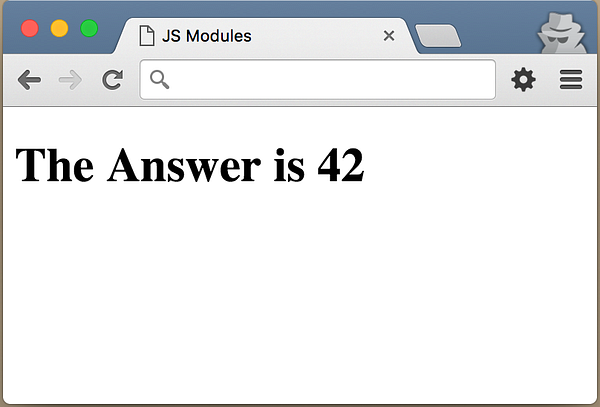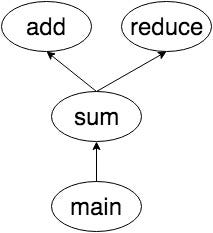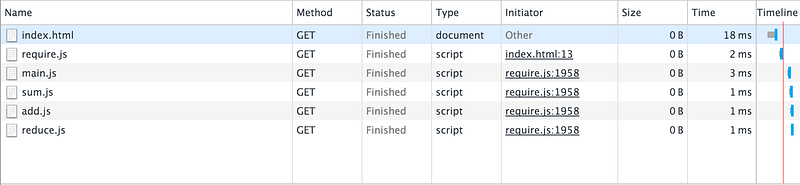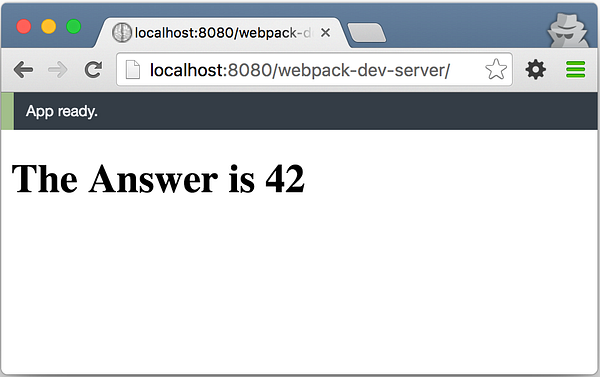# JavaScript 模块简史

• 网络埋伏纪事
• 5907 访问## 示例应用程序函数的依赖示意图

`main` 函数计算数组中数字的和，然后把答案显示给 `span#answer``sum` 函数依赖于两个函数：`add``reduce``add` 函数做它名字所做的，把两个数相加。`reduce` 函数遍历数组，并且调用 `iteratee` 回调函数。

0 - index.html

``````<!DOCTYPE html>
<html>
<meta charset="UTF-8">
<title>JS Modules</title>
<body>
<h1>
</h1>
</body>
</html>
``````

1 - main.js

``````var values = [ 1, 2, 4, 5, 6, 7, 8, 9 ];
``````

2 - sum.js

``````function sum(arr){
}
``````

``````function add(a, b) {
return a + b;
}
``````

4 - reduce.js

``````function reduce(arr, iteratee) {
var index = 0,
length = arr.length,
memo = arr[index];
for(index += 1; index < length; index += 1){
memo = iteratee(memo, arr[index])
}
return memo;
}
``````

## 使用内嵌脚本

• 缺乏代码可重用性：如果需要添加另一个页面，并需要本页上的一些功能，我们就不得不复制粘贴代码。
• 缺乏依赖解析：你必须保证 `main` 函数之前就有 `add``reduce``sum` 函数。
• 命名空间污染：所有的函数诶变量将都驻留在全局作用域。
``````<!-- index.html -->

<!DOCTYPE html>
<html>
<meta charset="UTF-8">
<title>JS Modules</title>
<body>
<h1>
</h1>

<script type="text/javascript">
return a + b;
}
function reduce(arr, iteratee) {
var index = 0,
length = arr.length,
memo = arr[index];
for(index += 1; index < length; index += 1){
memo = iteratee(memo, arr[index])
}
return memo;
}
function sum(arr){
}
/* Main Function */
var values = [ 1, 2, 4, 5, 6, 7, 8, 9 ];
</script>
</body>
</html>
``````

## 使用`Script` 标记链接外部 JavaScript 文件

• 缺乏依赖解析：文件的顺序很重要。你要负责在 main.js 文件之前包含 `add.js``reduce.js``sum.js` 文件。
• 全局命令空间污染：所有的函数和变量依然在全局作用域中。

0 - index.html

``````<!DOCTYPE html>
<html>
<meta charset="UTF-8">
<title>JS Modules</title>
<body>
<h1>
</h1>

<script type="text/javascript" src="./reduce.js"></script>
<script type="text/javascript" src="./sum.js"></script>
<script type="text/javascript" src="./main.js"></script>
</body>
``````

``````//add.js
return a + b;
}
``````

2 - reduce.js

``````//reduce.js
function reduce(arr, iteratee) {
var index = 0,
length = arr.length,
memo = arr[index];

index += 1;
for(; index < length; index += 1){
memo = iteratee(memo, arr[index])
}
return memo;
}
``````

3 - sum.js

``````//sum.js
function sum(arr){
}
``````

4 - main.js

`````` // main.js
var values = [ 1, 2, 4, 5, 6, 7, 8, 9 ];
``````

## 模块对象和 IIFE（模块模式）

``````// 01  my-app.js
var myApp = {};
``````
``````// 02 - add.js
(function(){
return a + b;
}
})();
``````
``````// 03 - reduce.js
(function(){
myApp.reduce = function(arr, iteratee) {
var index = 0,
length = arr.length,
memo = arr[index];

index += 1;
for(; index < length; index += 1){
memo = iteratee(memo, arr[index])
}
return memo;
}
})();
``````
``````// 04 - sum.js
(function(){
myApp.sum = function(arr){
}
})();
``````
``````// 05 - main.js
(function(app){
var values = [ 1, 2, 4, 5, 6, 7, 8, 9 ];
})(myApp);
``````
``````// 06 - index.html
<!DOCTYPE html>
<html>
<meta charset="UTF-8">
<title>JS Modules</title>
<body>
<h1>
</h1>

<script type="text/javascript" src="./my-app.js"></script>
<script type="text/javascript" src="./reduce.js"></script>
<script type="text/javascript" src="./sum.js"></script>
<script type="text/javascript" src="./main.js"></script>
</body>
</html>
``````

``````(function(){ /*... your code goes here ...*/ })();
``````

``````myApp.add(1,2);
myApp.sum([1,2,3,4]);
``````

``````(function(obj){
// obj 是一个新的 veryLongNameOfGlobalObject
})(veryLongNameOfGloablObject);
``````

• 缺乏依赖解析：文件的顺序依然重要，`myApp.js` 必须出现在所有其它文件之前，`main.js` 必须处在所有其它库文件之后。
• 全局命令空间污染：现在全局变量的数量变成了 1，但是还不是 0 。

## CommonJS

CommonJS 并非一个 JavaScript 库，而是一个标准化组织，像 ECMA 或者 W3C 一样。ECMA 定义了 JavaScript 语言规范。W3C 定义了 JavaScript Web API，比如 DOM 和 DOM 事件。CommonJS 的目标是为 Web 服务器、桌面和命令行应用程序定义一套通用的 API。

CommonJS 还定义了模块 API 。因为在服务器应用程序中没有 HTML 页面，也没有 script 标记，所以就得有一些清晰的模块 API。模块需要暴露（export）给其它模块使用，并且是可访问的（import）。它的输出模块语法像这样的：

``````// add.js
return a+b;
}
``````

``````var add = require(‘./add’);
``````

## AMD（异步模块定义）

CommonJS 风格的模块定义的问题是，它不是异步的。当调用 `var add=require(‘add’);`时，系统会暂停，直到模块准备好了。这意味着在所有模块正在加载时，这行代码会冻结浏览器。所以这可能不是定义浏览器端模块的最佳方式。 为了把服务器端用的模块语法转换给浏览器端用，CommonJS 提出了几种模块格式。其中之一，即 “Module/Transfer/C“，后来成为异步模块定义（AMD）。

AMD 的格式如下：

``````define([‘add’, ‘reduce’], function(add, reduce){
return function(){...};
});
``````

`define` 函数（或者关键字）用依赖列表和一个回调函数做参数。回调函数的参数与数组中的依赖有相同的次序。这等于导入模块。而回调函数会返回一个值，该值就是输出的值。

CommonJS 和 AMD 解决了模块模式剩下的两个问题：依赖解析全局作用域污染，现在我们只需要注意每个模块或者文件的依赖，并且不再有全局作用域污染。

## RequireJS

AMD 可以把我们从浏览器应用程序中的 script 标记黑洞和全局污染中解救出来。那么，我们该如何使用它呢？这里 RequireJS 就可以帮助我们了。RequireJS 是一个 JavaScript 模块加载器。它可以帮助我们按需异步加载模块。

AMD 风格的示例应用程序

0 - index.html

``````<!DOCTYPE html>
<html>
<meta charset="UTF-8">
<title>JS Modules</title>
<body>
<h1>
</h1>

<script data-main="main" src="require.js"></script>
</body>
</html>
``````

1 - main.js

``````// main.js
define(['sum'], function(sum){
var values = [ 1, 2, 4, 5, 6, 7, 8, 9 ];
})
``````

2 - sum.js

``````// sum.js
var sum = function(arr){
};

return sum;
})
``````

``````// add.js
define([], function(){
return a + b;
};

});
``````

4 - reduce.js

``````// reduce.js
define([], function(){
var reduce = function(arr, iteratee) {
var index = 0,
length = arr.length,
memo = arr[index];

index += 1;
for(; index < length; index += 1){
memo = iteratee(memo, arr[index])
}
return memo;
}

return reduce;
})
``````

``````<script data-main="main" src="require.js"></script>
``````RequireJS 和 AMD 解决了我们以前所遇到的所有问题。但是，它有带来了其它一些不怎么严重的问题：

• AMD 语法很古怪。因为所有东西都封装在 `define` 函数内，代码就有一些额外的缩进。对于小文件来说，这不是啥问题，但是对于大的代码库来说，就可能是精神上的疲惫。
• 数组中的依赖列表必须与函数的参数列表匹配。如果有很多依赖，就很难维护依赖的次序。如果模块中有几十个依赖，后来又要从中间删除一个，那么就很难找到匹配的模块和参数。
• 在当前浏览器下（HTTP 1.1），加载很多小文件会降低性能。

## Browserify

RequireJS 是一个 JS 库，但是 Browserify 是一个命令行工具，需要 NodeJS 和 NPM 来按住那个它。如果系统中安装了 NodeJS，就可以用如下命令来安装 Browserify：

``````npm install -g browserify
``````

0 - index.html

``````<!DOCTYPE html>
<html>
<meta charset="UTF-8">
<title>JS Modules</title>
<body>
<h1>
</h1>

<script src="bundle.js"></script>
</body>
</html>
``````

1 - main.js

``````//main.js
var sum = require('./sum');
var values = [ 1, 2, 4, 5, 6, 7, 8, 9 ];

``````

2 - sum.js

``````//sum.js
var reduce = require('./reduce');

module.exports = function(arr){
};
``````

``````//add.js
return a + b;
};
``````

4 - reduce.js

``````//reduce.js
module.exports = function reduce(arr, iteratee) {
var index = 0,
length = arr.length,
memo = arr[index];

index += 1;
for(; index < length; index += 1){
memo = iteratee(memo, arr[index])
}
return memo;
};
``````

``````\$ brwoserify main.js -o bundle.js
``````

Browserify 解析 `main.js` 中的 `require()` 函数调用，并遍历项目中的依赖树。然后将依赖树打包到一个文件中。

``````function e(t,n,r){function s(o,u){if(!n[o]){if(!t[o]){var a=typeof require=="function"&&require;if(!u&&a)return a(o,!0);if(i)return i(o,!0);var f=new Error("Cannot find module '"+o+"'");throw f.code="MODULE_NOT_FOUND",f}var l=n[o]={exports:{}};t[o].call(l.exports,function(e){var n=t[o][e];return s(n?n:e)},l,l.exports,e,t,n,r)}return n[o].exports}var i=typeof require=="function"&&require;for(var o=0;o<r.length;o++)s(r[o]);return s})({1:[function(require,module,exports){
return a + b;
};

},{}],2:[function(require,module,exports){
var sum = require('./sum');
var values = [ 1, 2, 4, 5, 6, 7, 8, 9 ];

},{"./sum":4}],3:[function(require,module,exports){
module.exports = function reduce(arr, iteratee) {
var index = 0,
length = arr.length,
memo = arr[index];

index += 1;
for(; index < length; index += 1){
memo = iteratee(memo, arr[index])
}
return memo;
};

},{}],4:[function(require,module,exports){
var reduce = require('./reduce');

module.exports = function(arr){
};

``````

## UMD — 只会让你更糊涂

``````//sum.umd.js
(function (root, factory) {
if (typeof define === 'function' && define.amd) {
// AMD
} else if (typeof exports === 'object') {
// Node, CommonJS-like
} else {
// Browser globals (root is window)
}
// private methods

// exposed public methods
return function(arr) {
}
}));
``````

## ES6 模块语法

JavaScript 全局模块对象、CommonJS、AMD 和 UMD，太多选择了。现在也许你会问，下一个项目我该用哪一个呢？答案是一个都不用。

JavaScript 语言中并没有内置模块系统。这正是我们有如此多输入和输出模块的不同方式的原因。但是这种情况最近得到改变了。在 ES6 规范中，模块已经成为 JavaScript 的一部分。所以这个问题的答案是，如果想让项目不会过时，就得用 ES6 模块语法。

ES6 用 `import``export` 关键字来输入和输出模块。如下是用 ES6 模块语法编写的示例应用程序。

01 - main.js

``````// main.js
import sum from "./sum";

var values = [ 1, 2, 4, 5, 6, 7, 8, 9 ];

``````

02 - sum.js

``````// sum.js
import reduce from './reduce';

export default function sum(arr){
}
``````

``````// add.js
return a + b;
}
``````

04 - reduce.js

``````//reduce.js
export default function reduce(arr, iteratee) {
let index = 0,
length = arr.length,
memo = arr[index];

index += 1;
for(; index < length; index += 1){
memo = iteratee(memo, arr[index]);
}
return memo;
}
``````

## Webpack

Webpack 是一个模块打包器。就像 Browserify 一样，它会遍历依赖树，然后将其打包到一到多个文件。如果 Webpack 与 Browserify 完全相同，那么我们为什么依然需要另一个模块打包器呢？Webpack 可以处理CommonJS、AMD 和 ES6 模块，并且它带来了更大的灵活性和很酷的一些功能，比如：

• 代码分离：如果有多个 app 共享相同的模块。Webpack 可以将代码打包到两到多个文件。例如，如果有两个 app：app1 和 app2，二者都共用很多模块。如果用 Browserify 的话，就有 app1.js 和 app2.js，两个文件都要包含所有依赖模块。但是如果是用 Webpack 的话，我们就可以创建 app1.js、app2.js 和 shared-lib.js。是的，我们必须从 html 页面中加载两个文件。但是有了哈希文件名、浏览器缓存和 CDN，就可以降低初始加载时间。
• 加载器：用自定义加载器，可以加载任何文件到源文件中。用 `require()` 语法，不仅仅可以加载 JavaScript 文件，还可以加载 CSS、CoffeeScript、Sass、Less、HTML模板、图像，等等。
• 插件：Webpack 插件可以在打包写入到文件之前对它进行操作。有很多社区创建的插件。例如，给打包代码添加注释，添加 Source map，将打包文件分离成块等等。
• WebpackDevServer 是一个开发服务器，它可以在源代码改变被检测到时自动打包源代码，并刷新浏览器。通过提供代码的即时反馈，从而加快开发过程。

``````\$ mkdir project; cd project
\$ npm init -y
\$ npm install -D webpack webpack-dev-server
``````

``````module.exports = {
entry: ‘./app/main.js’,
output: {
filename: ‘bundle.js’
}
}
``````

``````"scripts": {
"start": "webpack-dev-server -progress -colors",
"build": "webpack"
},
``````

01 - index.html

``````<!DOCTYPE html>
<html>
<meta charset="UTF-8">
<title>JS Modules</title>
<body>
<h1>
</h1>

<script src="bundle.js"></script>
</body>
</html>
``````

02 - webpack.config.js

``````module.exports = {
entry: './app/main.js',
output: {
path: './dist',
filename: 'bundle.js'
}
}
``````

03 - package.json

``````{
"name": "jsmodules",
"version": "1.0.0",
"description": "",
"main": "main.js",
"scripts": {
"start": "webpack-dev-server --progress --colors",
"build": "webpack"
},
"keywords": [],
"author": "",
"devDependencies": {
"webpack": "^1.12.14",
"webpack-dev-server": "^1.14.1"
}
}
``````

``````// app/add.js
return a + b;
};
``````

05 - app-reduce.js

``````// app/reduce.js
module.exports = function reduce(arr, iteratee) {
var index = 0,
length = arr.length,
memo = arr[index];

index += 1;
for(; index < length; index += 1){
memo = iteratee(memo, arr[index])
}
return memo;
};
``````

06 - app-sum.js

``````// app/sum.js
sum =  function(arr){
}

return sum;
});
``````

07 - app-main.js

``````// app/main.js
var sum = require('./sum');
var values = [ 1, 2, 4, 5, 6, 7, 8, 9 ];

``````

``````\$ npm start
``````webpack dev server in action

``````\$ npm run build
``````

## Rollup (2015 年 5 月)

Rollup 可以被用作为命令行工具。如果有 NodeJS 和 NPM，那么就可以用如下命令安装 rollup：

``````\$ npm install -g rollup
``````

Rollup 可以与任何类型的模块风格一起工作。但是，推荐使用 ES6 模块风格，这样就可以利用 tree-shaking 功能。如下是用 ES6 编写的示例应用程序代码：

``````let add = (a,b) => a + b;
let sub = (a,b) => a - b;

``````

02 - reduce.js

``````// reduce.js
export default (arr, iteratee) => {
let index = 0,
length = arr.length,
memo = arr[index];

index += 1;
for(; index < length; index += 1){
memo = iteratee(memo, arr[index]);
}
return memo;
}
``````

03 - sum.js

``````// sum.js
import reduce from './reduce';

export default (arr) => reduce(arr, add);
``````

04 - main.js

``````// main.js
import sum from "./sum";

var values = [ 1, 2, 4, 5, 6, 7, 8, 9 ];

``````

``````\$ rollup main.js -o bundle.js
``````

``````let add = (a,b) => a + b;

var reduce = (arr, iteratee) => {
let index = 0,
length = arr.length,
memo = arr[index];

index += 1;
for(; index < length; index += 1){
memo = iteratee(memo, arr[index]);
}
return memo;
}

var sum = (arr) => reduce(arr, add);

var values = [ 1, 2, 4, 5, 6, 7, 8, 9 ];

``````

## SystemJS

SystemJS 是一个通用的模块加载器，它能在浏览器或者 NodeJS 上动态加载模块，并且支持 CommonJS、AMD、全局模块对象和 ES6 模块。通过使用插件，它不仅可以加载 JavaScript，还可以加载 CoffeeScript 和 TypeScript。

SystemJS 的另一个优点是，它建立在 ES6 模块加载器之上，所以它的语法和 API 在将来很可能是语言的一部分，这会让我们的代码更不会过时。

``````System.import(‘module-name’);
``````

``````System.config({
transplier: ‘babel’,
baseURL: ‘/app’
});
``````

## JSPM

JSPM 是 JavaScript 开发工具的瑞士军刀，它是既是包管理器，又是模块加载器，又是模块打包器。

``````jspm install npm:package-name or github:package/name
``````

``````jspm bundle main.js bundle.js
``````

## 总结